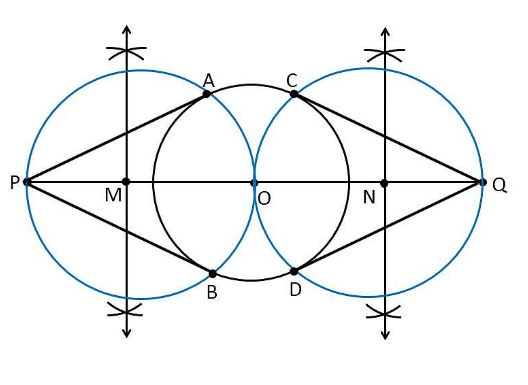Guru

# Draw a circle of radius 3 cm. Take two points P and Q on one of its extended diameter each at a distance of 7 cm from its centre. Draw tangents to the circle from these two points P and Q Q.3

• 0

Sir give me the best way to solve the problem of Construction chapter of exercise 11.2 of ncert class 10th math of question no.3, how i solve this question in simple and easy way Draw a circle of radius 3 cm. Take two points P and Q on one of its extended diameter each at a distance of 7 cm from its centre. Draw tangents to the circle from these two points P and Q

Share

1. Construction Procedure:

The tangent for the given circle can be constructed as follows.

1. Draw a circle with a radius of 3cm with centre “O”.

2. Draw a diameter of a circle and it extends 7 cm from the centre and mark it as P and Q.

3. Draw the perpendicular bisector of the line PO and mark the midpoint as M.

4. Draw a circle with M as centre and MO as radius

5. Now join the points PA and PB in which the circle with radius MO intersects the circle of circle 3cm.

6. Now PA and PB are the required tangents.

7. Similarly, from the point Q, we can draw the tangents.

8. From that, QC and QD are the required tangents.Justification:

The construction of the given problem can be justified by proving that PQ and PR are the tangents to the circle of radius 3 cm with centre O.

To prove this, join OA and OB.

From the construction,

∠PAO is an angle in the semi-circle.

We know that angle in a semi-circle is a right angle, so it becomes,

∴ ∠PAO = 90°

Such that

⇒ OA ⊥ PA

Since OA is the radius of the circle with radius 3 cm, PA must be a tangent of the circle. Similarly, we can prove that PB, QC and QD are the tangent of the circle.

Hence, justified

• 0